### Home > CALC > Chapter 1 > Lesson 1.3.2 > Problem1-120

1-120.
1. A flag in the shape of a quarter-circle is shown below. Homework Help ✎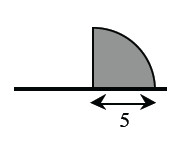1. Imagine rotating the flag about its pole and describe the resulting three-dimensional figure. Draw a picture of this figure on your paper. Try this using the 1-120 eTool (Desmos).

2. Find the volume of the rotated flag.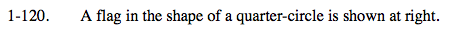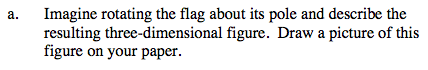What three-dimensional figure does this shape remind you of?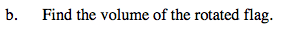What is the formula for the volume of a sphere?

How would you determine the volume of half of a sphere?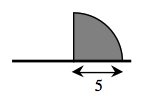Use the eTool below to visualize the rotating flag.
Click the link at right for the full version of the eTool: Calc 1-120 HW eTool